#### STAT 450

Problems: Assignment 6

1. Page 365. Q 21.

2. Page 365 Q 24.

3. Page 366. Q 28.

4. Page 366. Q 29.

5. Suppose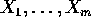are iid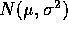and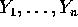are iid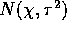.

1. Find complete and sufficient statistics.

2. Find UMVUE's of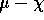and.

3. Now suppose you know that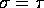. Find UMVUE's of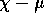and of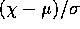. (You have already found the UMVUE for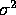.)

4. Now suppose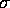and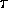are unknown but that you know that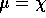. Prove there is no UMVUE for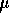. (Hint: Find the UMVUE if you knew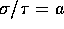with a known. Use the fact that the solution depends on a to finish the proof.)

5. Why doesn't the Lehmann-Scheffé theorem apply?

6. Suppose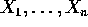iid Poisson(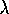). Find the UMVUE forand for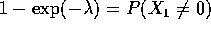.

7. Let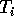be the error sum of squares in the ith cell in the question 5 of Assignment 5.

1. Find the joint density of the.

2. Find the best estimate ofof the form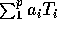in the sense of mean squared error.

3. Do the same under the condition that the estimator must be unbiased.

4. If only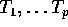are observed what is the MLE of?

5. Find the UMVUE offor the usual one-way layout model, that is, the model of the last two questions.

8. Exponential families: Supposeare iid with density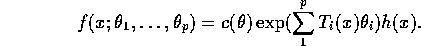1. Find minimal sufficient statistics.

2. If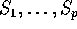are the minimal sufficient statistics show that setting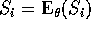and solving gives the likelihood equations. (Note the connection to the method of moments.)

Richard Lockhart
Sun Oct 27 19:00:53 PST 1996